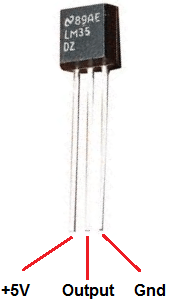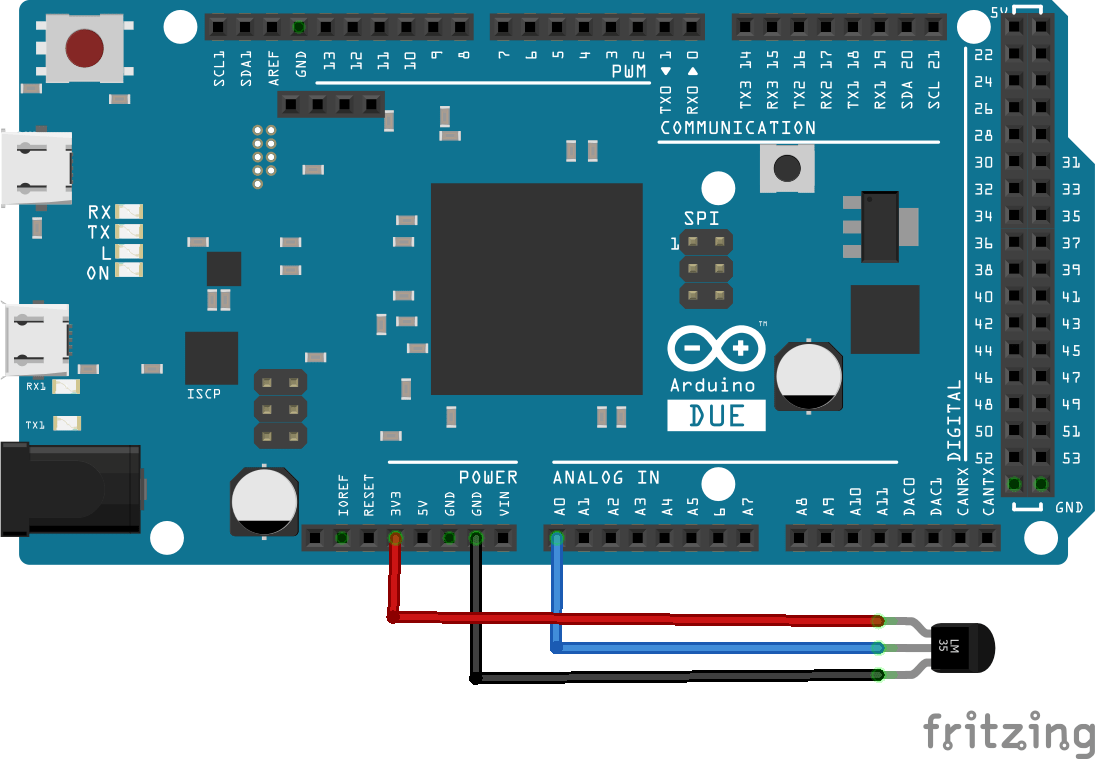# Arduino Due and LM35 temperature sensor

In this example we will connect an LM35 temperature sensor to our Arduino Due</p><p>The LM35 series are precision integrated-circuit temperature sensors, whose output voltage is linearly proportional to the Celsius (Centigrade) temperature. The LM35 thus has an advantage over linear temperature sensors calibrated in Kelvin, as the user is not required to subtract a large constant voltage from its output to obtain convenient Centigrade scaling. The LM35 does not require any external calibration or trimming to provide typical accuracies of ±1/4°C at room temperature and ±3/4°C over a full -55 to +150°C  temperature range

Here is a picture of the pins, its important to get these correct or you can damage the sensorSchematics

Very simple to connect

Vcc is 3v3
Gnd is any Gnd and out goes to Arduino A0, you can see this belowCode

[codesyntax lang=”cpp”]

```const int analogIn = A0;

int RawValue= 0;
double Voltage = 0;
double tempC = 0;
double tempF = 0;

void setup(){
Serial.begin(9600);
}

void loop(){

Voltage = (RawValue / 1023.0) * 3300; // 5000 to get millivots.
tempC = Voltage * 0.1;
tempF = (tempC * 1.8) + 32; // conver to F
Serial.print("Raw Value = " ); // shows pre-scaled value
Serial.print(RawValue);
Serial.print("\t milli volts = "); // shows the voltage measured
Serial.print(Voltage,0); //
Serial.print("\t Temperature in C = ");
Serial.print(tempC,1);
Serial.print("\t Temperature in F = ");
Serial.println(tempF,1);
delay(500);
}```

[/codesyntax]

Output

Open the serial monitor

Raw Value = 68 milli volts = 219 Temperature in C = 21.9 Temperature in F = 71.5
Raw Value = 70 milli volts = 226 Temperature in C = 22.6 Temperature in F = 72.6
Raw Value = 71 milli volts = 229 Temperature in C = 22.9 Temperature in F = 73.2
Raw Value = 72 milli volts = 232 Temperature in C = 23.2 Temperature in F = 73.8
Raw Value = 73 milli volts = 235 Temperature in C = 23.5 Temperature in F = 74.4
Raw Value = 74 milli volts = 239 Temperature in C = 23.9 Temperature in F = 75.0
Raw Value = 75 milli volts = 242 Temperature in C = 24.2 Temperature in F = 75.5
Raw Value = 76 milli volts = 245 Temperature in C = 24.5 Temperature in F = 76.1
Raw Value = 77 milli volts = 248 Temperature in C = 24.8 Temperature in F = 76.7

5PCS LM35D Temperature Sensor TO92 Packing